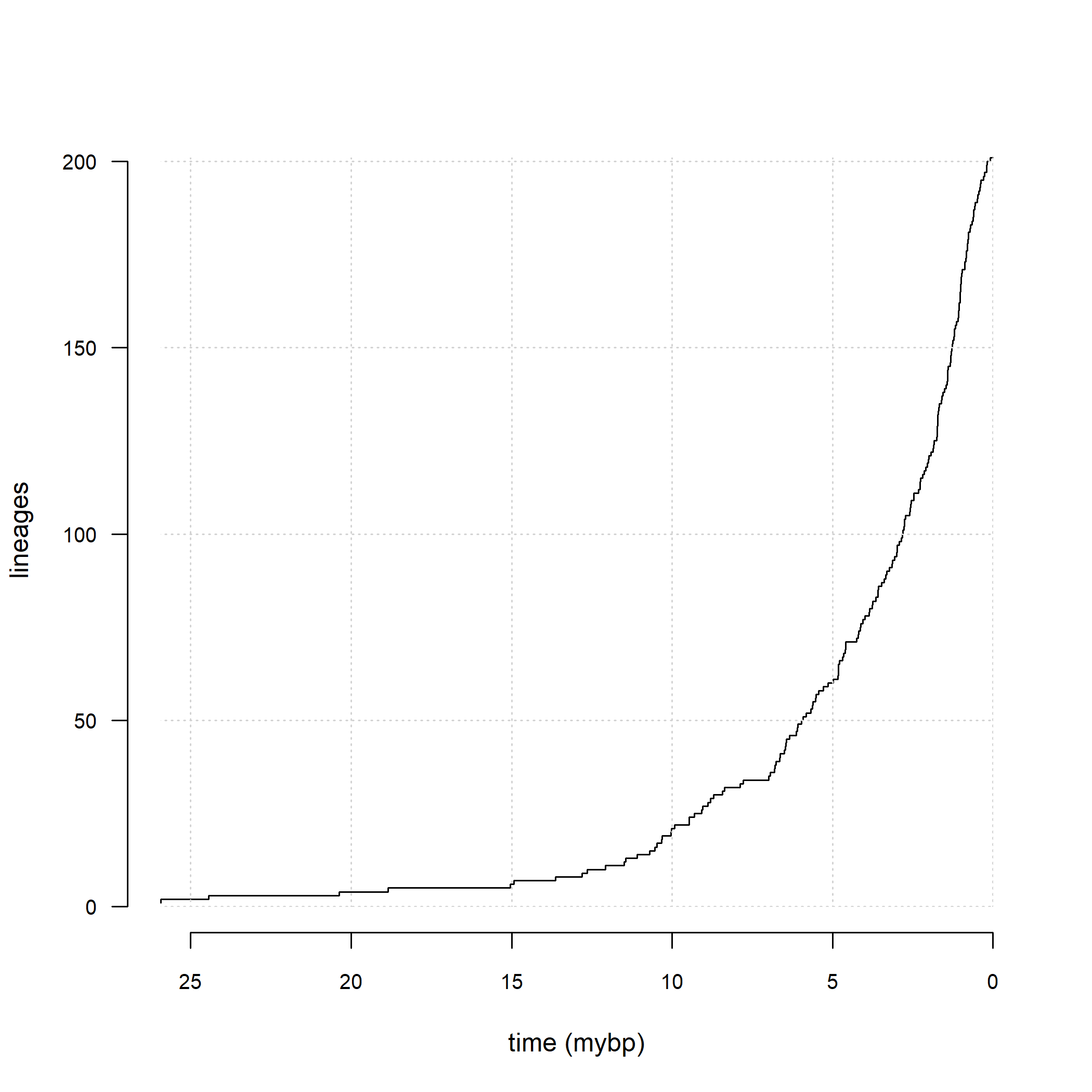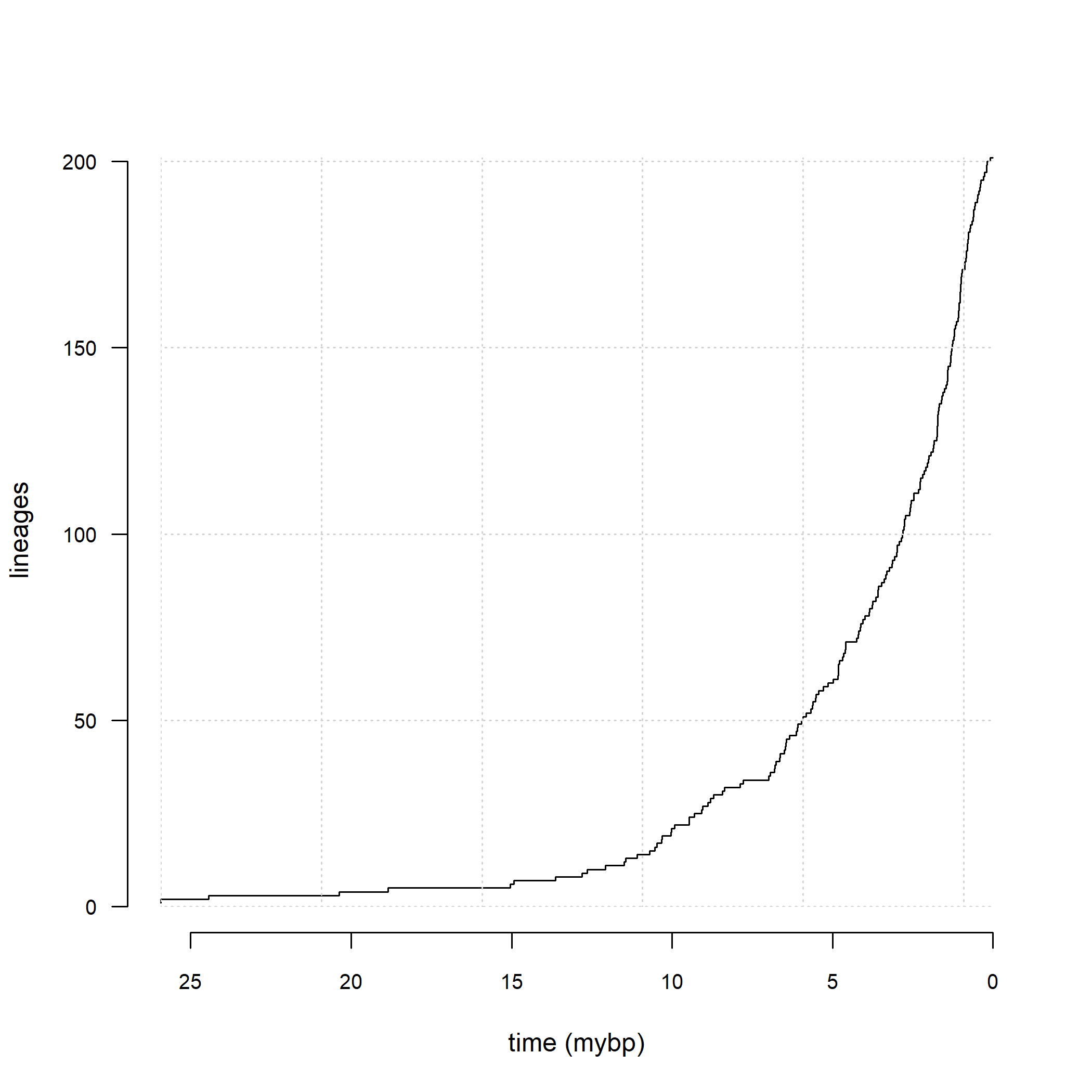## Thursday, August 4, 2022

### Two ways to flip our x-axis on a lineage-through-time plot

A colleague asked me if there was a way to flip the x-axis of a lineage-through-time plot, so that it runs backwards in time from 0 (the present day) to the depth of the root.

I actually recently demo-ed one solution on this blog. In that case, the way I did it was by first making the plot without an x-axis, and then adding the x-axis back in, but with the labels plotted in reverse order!

There's another, possibly easier, way to accomplish basically the same effect using graphical parameters function `par`.

Here's a quick illustration.

``````library(phytools)
darter.ltt<-ltt(darter.tree,plot=FALSE)
plot(darter.ltt,axes=FALSE,log.lineages=FALSE,
xlab="time (mybp)")
axis(2,las=2,cex.axis=0.8)
xaxp<-par()\$xaxp[c(2,1,3)]
par(usr=par()\$usr[c(2,1,3,4)])
par(xaxp=xaxp)
axis(1,cex.axis=0.8)
clip(x1=max(nodeHeights(darter.tree)),x2=0,y1=0,
y2=Ntip(darter.tree))
grid()
``````Just to review, here's how I flipped the axis in my prior post.

``````plot(darter.ltt,axes=FALSE,log.lineages=FALSE,
xlab="time (mybp)")
axis(2,las=2,cex.axis=0.8)
labs<-axTicks(1)
h<-max(nodeHeights(darter.tree))
at<-h-labs
axis(1,at=at,labels=labs,cex.axis=0.8)
clip(x1=0,x2=h,y1=0,
y2=Ntip(darter.tree))
grid()
``````See the difference? (Hint: look at the grid lines!)

That's it.